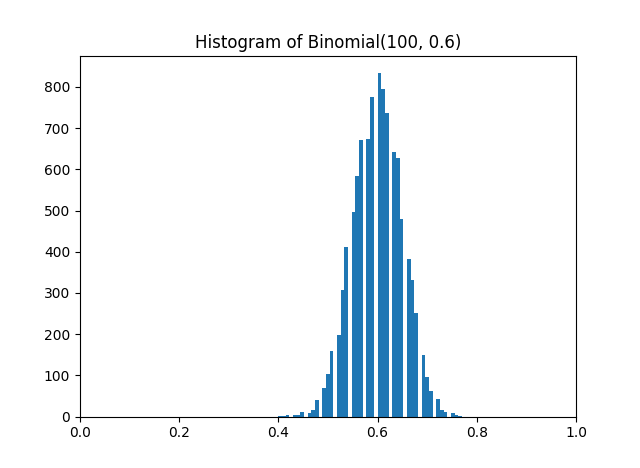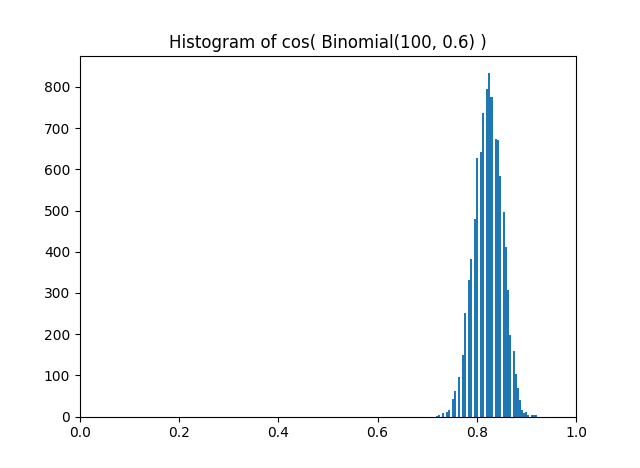# Applying probability to non-random things

Probability has surprising uses, including applications to things that absolutely are not random. I’ve touched on this a few times. For example, I’ve commented on how arguments about whether something is really random are often moot: Random is as random does.

This post will take non-random uses for probability in a different direction. We’ll start with discussion of probability and end up proving a classical analysis result.

Let p be the probability of success on some experiment and let Xn count the number of successes in n independent trials. The expected value of Xn is np, and so the expected value of Xn/n is p.

Now let be a continuous function on [0, 1] and think about f(Xn/n). As n increases, the distribution of Xn/n clusters more and more tightly around p, and so f(Xn/n) clusters more and more around f(p).

Let’s illustrate this with a simulation. Let p = 0.6, n = 100, and let f(x) = cos(x).

Here’s a histogram of random samples from Xn/n.And here’s a histogram of random samples from f(Xn/n). Note that it’s centered near cos(p) = 0.825.As n gets large, the expected value of f(Xn/n) converges to f(p). (You could make all this all rigorous, but I’ll leave out the details to focus on the intuitive idea.)

Now write out the expected value of f(Xn/n).Up to this point we’ve thought of p as a fixed probability, and we’ve arrived at an approximation result guided by probabilistic thinking. But the expression above is approximately f(p) regardless of how we think of it. So now let’s think of p varying over the interval [0, 1].

E[ f(Xn/n) ] is an nth degree polynomial in p that is close to f(p). In fact, as n goes to infinity, E[ f(Xn/n) ] converges uniformly to f(p).

So for an arbitrary continuous function f, we’ve constructed a sequence of polynomials that converge uniformly to f. In other words, we’ve proved the Weierstrass approximation theorem! The Weierstrass approximation theorem says that there exists a sequence of polynomials converging to any continuous function f on a bounded interval, and we’ve explicitly constructed such a sequence. The polynomials E[ f(Xn/n) ] are known as the Bernstein polynomials associated with f.

This is one of my favorite proofs. When I saw this in college, it felt like the professor was pulling a rabbit out of a hat. We’re talking about probability when all of a sudden, out pops a result that has nothing to do with randomness.

Now let’s see an example of the Bernstein polynomials approximating a continuous function. Again we’ll let f(x) = cos(x). If we plot the Bernstein polynomials and cosine on the same plot, the approximation is good enough that it’s hard to see what’s what. So instead we’ll plot the differences, cosine minus the Bernstein approximations.As the degree of the Bernstein polynomials increase, the error decreases. Also, note the vertical scale. Cosine of zero is 1, and so the errors here are small relative to the size of the function being approximated.

## 4 thoughts on “Applying probability to non-random things”

1. Very nice!!

2. SteveBrooklineMA

That is very nice! When the Bernstein Polynomials were presented to me in class in the proof of the Weierstrass Approximation theorem, they seemed to come out of nowhere. They worked, but how in the world did someone come up with this? The intuition provided in your post, on the other hand, is great.

What about uniformity of the approximation?

3. Very nice ! It looks like the kind of things that only Erdos could have invented.

4. Besides the elegance of the demonstration, this also serves to emphasize that probability mass functions and densities do not inherently rely on any sort of stochastic behavior. This a point I harass my statistics students on repeatedly, to help, I hope, prevent them from confusing the map (a distribution) with the territory (a random phenomenon). Thanks for outlining one of my newest future lectures!